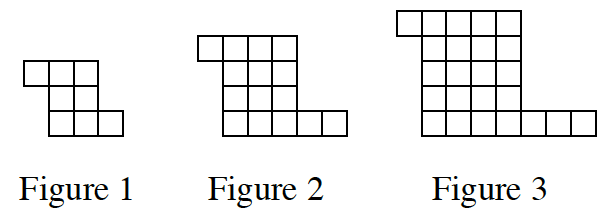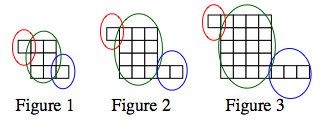Home > CCA > Chapter 9 > Lesson 9.1.4 > Problem9-40

9-40.

Find a rule that represents the number of tiles in Figure $x$ for the tile pattern below. Homework Help ✎The red circles point out the left arms,
the green circles point out the rectangles,
and the blue circles point out the right arms.

Notice the following:
The length of the rectangle in Figure 1 is 3 units × 2 units;
the rectangle in Figure 2 is 4 units × 3 units;
and the rectangle in Figure 3 is 5 units × 4 units.

First, look at the left arm. It remains constant in each of these figures, with a value of $1$.

Let $x=$ Figure number.
There is a rule for the size of the rectangles:
$(x+2)(x+1)$,
where $(x+2)$ represents the length
and $(x+1)$ represents the width.

Now, consider the right arms. Its length matches its corresponding figure number.

$y=$ left arm $+$ rectangle $+$ right arm
$y=1+(x+2)(x+1)+x$
Now simplify.